# Influence of Heat Source/Sink on Free Convection in Annular Porous Region

Influence of Heat Source/Sink on Free Convection in Annular Porous Region
Department of Mathematics, Institute of Science, Banaras Hindu University, Varanasi 221005, Uttar Pradesh, India

Department of Mathematics, Lok Nayak Jai Prakash Institute of Technology, Chhapra 841302, Bihar, India

Corresponding Author Email:
Page:
841-850
|
DOI:
https://doi.org/10.18280/ijht.390318
4 January 2020
|
Accepted:
26 December 2020
|
Published:
30 June 2021
| Citation

OPEN ACCESS

Abstract:

The significant interpretation of this model is to explore the influence of temperature-dependent heat source/sink on laminar free-convective flow in an annular porous region such as petroleum engineering, thermal technique and groundwater hydrology. For a unified solution of the Brinkman-Darcy model, the regulatory equations solved analytically by applying the variation of parameter technique in terms of Bessel's functions for the heat source and sink. Moreover, we have investigated the Variations of Darcy number, Heat source/sink and viscosity ratio in the presence of isothermal and constant heat flux sequentially. As a result, we received the critical value of the velocity for the radii ratio (R = 2.05 and 2.92) in both the cases of source and sink (S = 1.0 and Si = 0.1) respectively which is exhibited through the graphs. Further, the numerical outcomes present of the skin friction including volume flow with annular gap by the graphs as well as tables.

Keywords:

fully developed flow, natural convection, source and sink, isothermal and constant heat flux, modified Bessel function

1. Introduction

Studies of free convection between two vertical concentric cylinders filled with a saturated porous medium have been attracting the attention of Scientists and Engineers over the earlier decades. These domains have too much physical significance as it has many scientific and engineering applications such as petroleum engineering, groundwater hydrology, soil mechanics, engineering science, thermal technique, agriculture, and industries, etc. In free convection, fluid motion occurs by free means such as buoyancy. The natural convection happens due to the gravity of the earth on the heated fluid of density of the fluid and their respective buoyancy of the fluid. For more detailed concerning these applications the researchers can refer to the studies [1-7]. The increasing volume of the study in this zone has been extensively discussed by a number of books, like as, Ingham and Pop , Vafai , Pop and Ingham , Vadesz , and Nield and Bejan , Maurya et al.  and Oni . In porous media, most of the work related to convective heat transfer is based on Darcy’s law. The precision of these outcomes is constrained to a particular region of Darcy number and Reynolds number. Vafai and Tien  have been investigated the significance of Brinkman and Forchheimer's term over a flat plate in the presence of forced convection. They gave the resulting inaccuracy in the heat transfer coefficient when inertial and viscous terms are overlooked. Ranganathan and Viskanta  have handled the related investigation, and they got the effects of the wall and its inertia in mixed convective flow over a flattened plate.

Aung  has reported both plate and channel in fully evolved laminar convection flow while Nelson and wood  have solved the problem numerically for heat and mass transfer with free convection between vertical channels with symmetric boundary conditions of its temperature and concentration. Further, Joshi  has received the solution analytically for fully developed natural convection in an isothermal vertical tube and compares his result got by the finite-difference technique. Using Brinkman- extended Darcy model, Paul and Singh  have studied in detail the convection within coaxial vertical cylinders partly saturated with a porous material and found that the velocity is turned by the shear stress jump situation at the boundary. Tong and Subramanian , and Lauriat and Prasad  have shown that wall effect is not much dominant for Darcy number much lesser than 10-5 in free convection. They have also measured the no-slip boundary condition for flow in porous medium and the precision of the result obtained from a pure Darcy approach are verified. Parang and Keyhani  have studied boundary interest in the mixed convective flow through an annular porous medium. Their outcomes show that the circumstances under which the Brinkman term can be disregarded without ascertaining an unwanted error. Prasad et al.  have studied the free convection in a vertical porous annulus with uniform heat flux on the interior wall. The significance of this result reveals that the hypothetical design must be customized to cover the channeling impressions in the boundary zone. Havstad and Burns  have observed numerical solutions for the convective heat transferable in vertical cylindrical annuli packed with a porous medium. It has noticed that the Perturbation solutions are efficient in all cylindrical areas and low-temperature differentiation, while asymptotic solutions are proven for long cylinders and high-temperature differentials.

Muralidhar and Kulacki , and Kaviany  have discussed the non-Darcy effects of natural convection in porous media between horizontal cylinders. Later, Khanafer and Chamkha  have studied mixed convection within a porous heat generating horizontal annulus in detail by using the Galerkin method. They also show the effect of the annulus gap, the Darcy number and heat transfer analysis. Murty et al.  have shown that the impact of the radii ratio in natural convection in detail. Bejan and Tien  have attempted the natural convection in horizontal space, enclosed by two concentric cylinders with distinct end temperatures. Singh et al.  have explained the flow formation problem in the isothermal and constant heat flux on the velocity and temperature in a vertical annulus. The analytical exploration for laminar fully developed sticky incompressible and electrically conducting liquid in an annulus in which the wall of the interior cylinder is heated or cooled either isothermally or at a constant heat flux while the exterior cylinder is maintained at ambient temperature was considered . Rapits and Takhar  have shown the structure of the flow pattern by generalized Forchheimer’s model for polar fluid in a porous medium enclosed by an infinitely long erect plate. Cheng [34, 35] showed the variations of temperature dependent viscosity on the natural convection heat transfer from a horizontal isothermal cylinder of the elliptical cross section. Another study on heat formation from an isothermal cylinder has performed by Molla et al. . Yadav and Singh [37, 38] have deliberated in detail the analysis of entropy in the annular porous duct. Analyses of porous media flow in annular regions have also been reported by Jha .

In addition, Ravi et al. [37, 40] have presented the free convection of a polar fluid between two vertical walls with the temperature dependent source/sink. Further, the amazing study of polar fluid with source/sink in a vertical annulus is done by Singh . Also, the numerical implications of the skin-friction for isothermal and uniform heat flux cases are submitted in the tabular form. In the field of MHD, Kumar and Singh [42, 43] have deliberated the influences of heat source/sink as well as the induced magnetic field in vertical annuli by considering the natural convection. On the free convective boundary layer flow, Merkin  first saw the variation of Newtonian heating. The alternations of the skin-friction and Nusselt number on the fluid velocity with the induced magnetic field and the induced current density have been carried out by Kumar and Singh . Yadav and Singh [46, 47] have compared the Darcy and viscous dissipations everywhere of the channel in a fully developed horizontal porous channel. Dwivedi et al.  have observed that the velocity and temperature profiles increase as the value of constant point/line heat source parameter increases.

In this study, inspired by Jha , we explain the impact of source/sink parameters in the case of isothermal and constant heat flux on natural convective flow through a vertical cylinder filled with a porous medium assuming viscous and Darcy terms. We have received the solutions, in the cases of source and sink, of momentum and energy equations with proper boundary limitations for the velocity and the temperature field. Ultimately, utilizing the graphs and tables, we have shown the impacts of Darcy number, viscosity ratio, and source/sink parameter on the velocity and the temperature field profiles in the case of isothermal and uniform/constant heat flux as well as on skin friction and the mass flux.

2. Mathematical Analysis

We have admitted, in a vertical annulus region of infinite length saturated by the porous medium, the steady and fully evolved laminar natural convective flow. The r'-axis is along the radial direction while z'-axis is vertically upward, perpendicular to the radial direction as shown in Figure 1. The radius of inner cylinder is taken as ‘a’ while that of outer cylinder is ‘b’. In the existing physical situation, the outer surface of the internal cylinder is heated or cooled either isothermally or uniform heat flux whereas the inner surface of the outward cylinder is sustained at the fixed temperature inducing the happening of free convection in the annular zone. The subject has been different physical situation extensively discussed [29, 30, 37].

## 1.png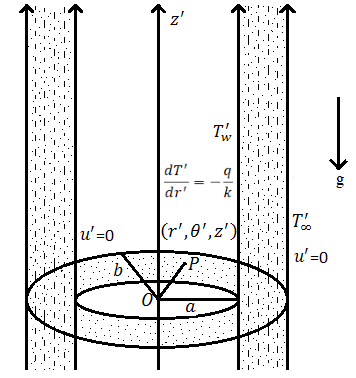Figure 1. Schematic diagram of the problem

As the flow has fully evolved and the expansion of the cylinder is endless, so the flow depends hardly on the coordinate r'. We have also taken the Boussinesq approximation and no slip condition in the existing problem.

Following these simplifying assumptions for free convective flow, the controlling differential equations in the dimensional forms is presented as:

${{\upsilon }_{eff}}\frac{1}{{{r}'}}\frac{d}{d{r}'}\left( {r}'\frac{d{u}'}{d{r}'} \right)-\frac{\upsilon {u}'}{{{K}'}}+g\beta \left( {T}'-{{{{T}'}}_{\infty }} \right)=0,$              (1)

$\frac{k}{\rho {{C}_{p}}}\frac{1}{{{r}'}}\frac{d}{d{r}'}\left( {r}'\frac{d{T}'}{d{r}'} \right)+\left( {T}'-{{{{T}'}}_{\infty }} \right)\frac{\Theta }{\rho {{C}_{p}}}=0.$      (2)

Under the above assumptions the boundary limitations for the velocity and temperature fields are:

$u^{\prime}=0, T^{\prime}=T_{w}^{\prime}$ or $\frac{d T^{\prime}}{d r^{\prime}}=-\frac{q}{k}$, at $r^{\prime}=a$,

$u^{\prime}=0, T^{\prime}=T_{\infty}^{\prime}$, at $r^{\prime}=b$.                              (3)

To simplify the problem, we have defined remarkable non-dimensional quantities as:

$r=\frac{r^{\prime}}{a}, R=\frac{b}{a}, D a=\frac{K^{\prime}}{a^{2}}, U=\frac{u^{\prime} v}{g \beta a^{2}\left(T_{w}^{\prime}-T_{\infty}^{\prime}\right)}$,

$T=\frac{T^{\prime}-T_{\infty}^{\prime}}{\Delta T}, M=\frac{v_{e f f}}{v}, S=\frac{\Theta a^{2}}{k}$                                   (4)

where, $\Delta T$ is $\left(T_{w}^{\prime}-T_{\infty}^{\prime}\right)$ or $\frac{q a}{k}$ according as the inner cylinder is sustained at a constant temperature $T_{W}^{\prime}$ or constant heat flux q.

By employing the above parameters, the controlling differential Eq. (1) and (2) communicating the model, in dimensionless form, are obtained as follows:

$M\frac{{{d}^{2}}U}{d{{r}^{2}}}+\frac{M}{r}\frac{dU}{dr}-\frac{U}{Da}=-T,$                       (5)

$\frac{{{d}^{2}}T}{d{{r}^{2}}}+\frac{1}{r}\frac{dT}{dr}+ST=0.$                       (6)

The relevant boundary limitations in non-dimensional form for corresponding to these equations are given as:

$U=0, T=1$ or $\frac{d T}{d r}=-1$, at $r=1$,                    (7)

$U=0, T=0$, at $r=R$.                   (8)

At the surface of the internal cylinder, the boundary limitations are a class of Dirichlet and Newmann. To achieve a novel result from together circumstances, the combined boundary conditions into a single form of the third kind are obtained as:

$a \frac{d T}{d r}+b T=c$ at $r=1$.                 (9)

The relevant values of the constants a, b and c will supply the isothermal or iso-flux condition on the external surface of the internal cylinder. The Eq. (5) and (6) with boundary limitations (8) and (9) are solved for the velocity and temperature profiles in both cases of source and sink.

2.1 When source is present

Heat source, it can exert a very huge amount of thermal energy without any change in its temperature. In the case of source parameter S>0, for which the velocity and the temperature expressions are:

\begin{align} & U={{C}_{2}}{{I}_{0}}\left( \frac{r}{\sqrt{DaM}} \right)+{{D}_{2}}{{K}_{0}}\left( \frac{r}{\sqrt{DaM}} \right) \\ & \quad \quad \quad +{{E}_{3}}\left[ {{C}_{1}}{{J}_{0}}\left( r\sqrt{S} \right)+{{D}_{1}}{{Y}_{0}}\left( r\sqrt{S} \right) \right], \\ \end{align}                       (10)

$T={{C}_{1}}{{J}_{0}}\left( r\sqrt{S} \right)+{{D}_{1}}{{Y}_{0}}\left( r\sqrt{S} \right).$                   (11)

To see the influence of velocity gradient at the boundaries, we obtained the skin-friction in non-dimensional at both boundaries in the terms of source, are given as:

\begin{align} & {{\tau }_{1}}={{\left( \frac{dU}{dr} \right)}_{r=1}} \\ & =\frac{1}{\sqrt{DaM}}\left[ {{C}_{2}}{{I}_{1}}\left( \frac{1}{\sqrt{DaM}} \right)-{{D}_{2}}{{K}_{1}}\left( \frac{1}{\sqrt{DaM}} \right) \right]-{{E}_{3}}{{F}_{1}}\sqrt{S}, \\ \end{align}                 (12)

\begin{align} & {{\tau }_{R}}=-{{\left( \frac{dU}{dr} \right)}_{r=R}} \\ & =-\frac{1}{\sqrt{DaM}}\left[ {{C}_{2}}{{I}_{1}}\left( \frac{R}{\sqrt{DaM}} \right)-{{D}_{2}}{{K}_{1}}\left( \frac{R}{\sqrt{DaM}} \right) \right]+{{E}_{3}}{{F}_{2}}\sqrt{S}. \\ \end{align}                (13)

In this case, the mass flux through the annular zone is obtained as follows:

$Q=2\pi \int\limits_{1}^{R}{rU\ dr}$

Q=2\pi \left\{ \begin{align} & {{C}_{2}}\sqrt{DaM}\left[ R{{I}_{1}}\left( \frac{R}{\sqrt{DaM}} \right)-{{I}_{1}}\left( \frac{1}{\sqrt{DaM}} \right) \right] \\ & -{{D}_{2}}\sqrt{DaM}\left[ R{{K}_{1}}\left( \frac{R}{\sqrt{DaM}} \right)-{{K}_{1}}\left( \frac{1}{\sqrt{DaM}} \right) \right] \\ & +\left( \frac{{{E}_{3}}{{C}_{1}}}{\sqrt{S}} \right)\left[ R{{J}_{1}}\left( R\sqrt{S} \right)-{{J}_{1}}\left( \sqrt{S} \right) \right] \\ & +\left( \frac{{{E}_{3}}{{D}_{1}}}{\sqrt{S}} \right)\left[ R{{Y}_{1}}\left( R\sqrt{S} \right)-{{Y}_{1}}\left( \sqrt{S} \right) \right] \\ \end{align} \right\}..                       (14)

2.2 When sink is present

When there is a temperature dependent sink, parameter S<0 and we take S=-Si, Si>0. In this case, the solutions for the velocity and the temperature field are in the terms of Si are obtained as:

\begin{align} & U={{A}_{2}}{{I}_{0}}\left( \frac{r}{\sqrt{DaM}} \right)+{{B}_{2}}{{K}_{0}}\left( \frac{r}{\sqrt{DaM}} \right) \\ & \quad \quad \quad -{{P}_{3}}\left[ {{A}_{1}}{{I}_{0}}\left( r\sqrt{Si} \right)+{{B}_{1}}{{K}_{0}}\left( r\sqrt{Si} \right) \right]\ , \\ \end{align}                (15)

$T={{A}_{1}}{{I}_{0}}\left( r\sqrt{Si} \right)+{{B}_{1}}{{K}_{0}}\left( r\sqrt{Si} \right).$                  (16)

To see the influence of velocity gradient at the boundaries, we obtained the skin-friction in non-dimensional at both boundaries in the terms of sink, are given as:

\begin{align} & {{\tau }_{1}}={{\left( \frac{dU}{dr} \right)}_{r=1}} \\ & =\frac{1}{\sqrt{DaM}}\left[ {{A}_{2}}{{I}_{1}}\left( \frac{1}{\sqrt{DaM}} \right)-{{B}_{2}}{{K}_{1}}\left( \frac{1}{\sqrt{DaM}} \right) \right]-{{P}_{3}}{{F}_{3}}\sqrt{Si}, \\ \end{align}                       (17)

\begin{align} & {{\tau }_{R}}=-{{\left( \frac{dU}{dr} \right)}_{r=R}} \\ & =-\frac{1}{\sqrt{DaM}}\left[ {{A}_{2}}{{I}_{1}}\left( \frac{R}{\sqrt{DaM}} \right)-{{B}_{2}}{{K}_{1}}\left( \frac{R}{\sqrt{DaM}} \right) \right]+{{P}_{3}}{{F}_{4}}\sqrt{Si}. \\ \end{align}                           (18)

In this case, the mass flux through the annular zone is obtained as follows:

$Q=2\pi \int\limits_{1}^{R}{rU\ dr}$

Q=2\pi \left\{ \begin{align} & {{A}_{2}}\sqrt{DaM}\left[ R{{I}_{1}}\left( \frac{R}{\sqrt{DaM}} \right)-{{I}_{1}}\left( \frac{1}{\sqrt{DaM}} \right) \right] \\ & -{{B}_{2}}\sqrt{DaM}\left[ R{{K}_{1}}\left( \frac{R}{\sqrt{DaM}} \right)-{{K}_{1}}\left( \frac{1}{\sqrt{DaM}} \right) \right] \\ & -\left( \frac{{{P}_{3}}{{A}_{1}}}{\sqrt{Si}} \right)\left[ R{{I}_{1}}\left( R\sqrt{Si} \right)-{{I}_{1}}\left( \sqrt{Si} \right) \right] \\ & +\left( \frac{{{P}_{3}}{{B}_{1}}}{\sqrt{Si}} \right)\left[ R{{K}_{1}}\left( R\sqrt{Si} \right)-{{K}_{1}}\left( \sqrt{Si} \right) \right] \\ \end{align} \right\}.              (19)

3. Results and Discussions

In the field of Physics, the transport phenomenon is understood and defined as a phenomenon that comprises of movement of a number of physical quantities that include energy, mass or momentum through either fluid or solid medium in existence of non-uniform conditions present within the medium. The diagrams in the situations of isothermal and constant heat flux with the source/sink are sketched to examine the outcomes of various controlling parameters like as Darcy number (Da) and viscosity ratio M on the velocity U and the temperature field T. The skin-friction τ, as well as the mass flow rate Q in their numerical values, is displayed in the tables for distinct values of the above parameters regulating the flow.

The temperature field sketches for the isothermal and uniform heat flux circumstances with source/sink have been depicted in Figure 2. We see from this figure that the temperature is more leading in the case of constant heat flux (Figure 2a) than the isothermal condition (Figure 2b) for the various values of the source (S=0.6, 0.9, 1.2) while the opposite appearance happens in the case of the sink (Si=0.6, 0.9, 1.2). Also from Figure 2a reveals that enhancement in heat source enhances the temperature. This is because the heat source adds extra heat to the annuli, which enhances its temperature. Due to this fact, it raises the thickness of the thermal boundary layer. Further, when heat sink (Figure 2b) enhances more heat deflected from the annuli, which diminish the thickness of the thermal boundary layer. This decreases the temperature profiles.

## 2a.png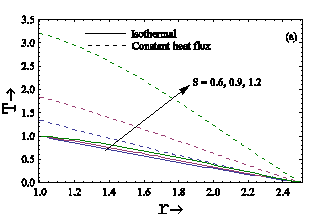## 2b.png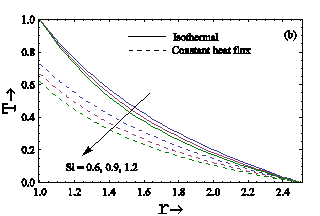Figure 2. Variation of the temperature field profiles at R=2.5 (a) for different values of Source parameter and (b) for different values of Sink parameter

From Figures 3-7, the velocity profiles have been drawn in both isothermal and constant heat flux cases for different values of Darcy number, the ratio of viscosity parameter and source/sink parameters. In both isothermal and constant heat flux cases, it is interpreted from Figure 3 that the fluid velocity progresses when the gap between the cylinders rises for both isothermal and constant heat flux cases.

## 3a.png## 3b.png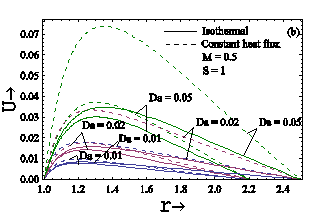## 3c.png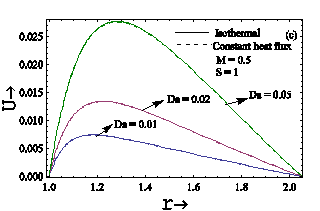## 3d.png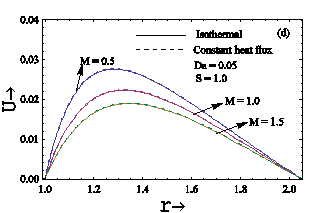Figure 3. Velocity sketches in the case of Source for (a) R<2.05 (b) R>2.05 (c) R=2.05 and (d) different value of M at R=2.05

Viewing these conclusions, it is a subject of attention to reach the critical value of R at which the velocity field sketches are nearly the same in the case of isothermal and uniform heat flux. It is found that the characteristics of the velocity field profiles of the fluid are almost identical in the case of isothermal and uniform heat flux when the critical value is R=2.05 in the case of source (S=1.0) (Figure 3). In the case of source, when the value of, the velocity is higher in isothermal case compared to the uniform heat flux case, while the reverse aspect occurs when four different values of Darcy number. For R=2.05, the velocity grows equally for rising values of Darcy number in the cases of isothermal and uniform heat flux while reverse phenomenon occurs in the Viscosity ratio parameter.

## 4a.png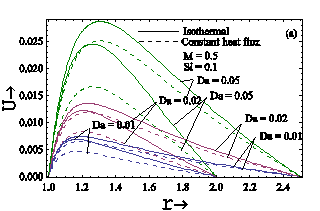## 4b.png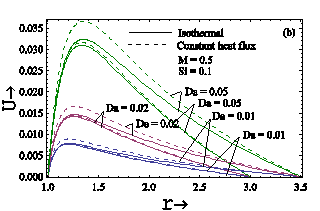## 4c.png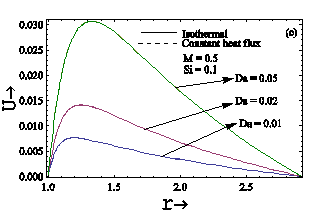## 4d.png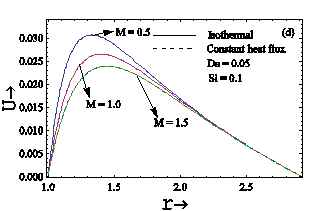Figure 4. Velocity sketches in the case of Sink for (a) R<2.92 (b) R>2.92 and (c) R=2.92 and (d) different value of M at R=2.92

In the case of sink (Figure 4), for the value of R<2.92, the velocity is greater for isothermal case compared to the constant heat flux case, while opposite phenomenon occurs when R>2.92 for different values of Darcy number. For R=2.92, the velocity field profiles are almost same for together cases of isothermal and constant heat flux with separate values of Darcy number and Viscosity ratio parameter in presence of sink (Si=0.1).

## 5a.png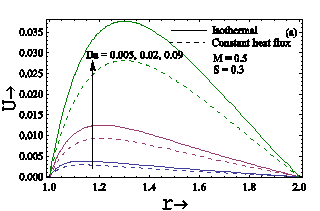## 5b.png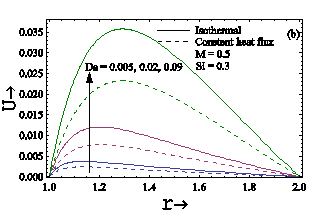Figure 5. Velocity sketches with Darcy number at R=2.0. (a) for source and (b) for sink

## 6a.png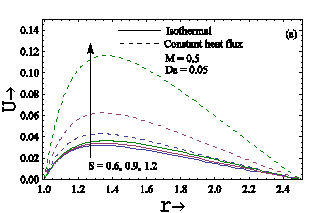## 6b.png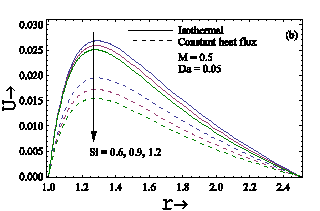Figure 6. Variation of the velocity profiles at R=2.5. (a) with different values of source and (b) with different values of sink

## 7a_2.png## 7b.png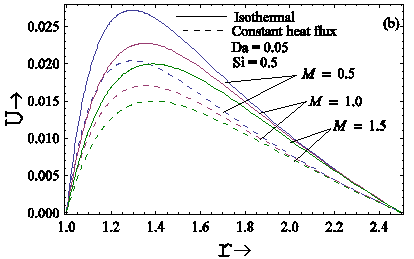Figure 7. Velocity sketches with various values of M at R=2.5. (a) for source and (b) for sink

The effects of Darcy number in the case of isothermal and constant heat flux, are shown in the Figure 5 and we observed that velocity rises with Darcy number. It means that the velocity has a linear relation with the Darcy number. The physical reason behind this is that the fluid velocity is elaborated by raising the permeability of the porous media and it is nearly equal to the Darcy number for a small value of the radius of the cylinder.

The effect of source/sink in the case of isothermal and constant heat flux are shown in the Figure 6. It is clear from the Figure 6 that velocity of the fluid increases in the case of source while it reduces in the sink. Thus, we can conclude that fluid velocity rises with increment in heat source parameter because the internal heat energy increases the thickness of the thermal boundary layer and the reverse phenomenon occur for the parameter of heat sink because the existence of sink absorbs the thermal energy which diminishes the velocity. Further, the effect of viscosity ratio parameters is to decrease the velocity field profiles in the case of isothermal and constant heat flux are shown in Figure 7. This sketch also shows the formation of boundary layers and the thickness of the boundary layer reduces as the value of 'M' rises due to the decrement in the viscous term.

Since we have taken no slip conditions at the boundaries, and the outer surface area is higher compare to inner surface area, therefore from Figures 3-7, we observed that the shearing stress is high near the outer surface compare to inner surface. So, velocity near the inner surface is high compare to the outer surface.

The effects of source/sink on skin-friction are presented in the Figures 8 and 9. Moreover, the effect of source/sink on the volume flow rate in the case of isothermal and constant heat flux is shown in the Figure 10. From these Figures we conclude that the skin friction and as well as dimensionless volume flow rate has shown the expanding look for the source while for sink the reversed action happens. In the attendance of source and sink, the skin friction and the volume flow rate is slightly change in the isothermal compared to the constant heat flux.

## 8a.png## 8b.png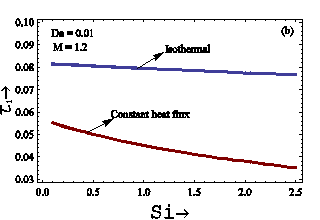Figure 8. Skin-friction of the internal cylinder at R=2.0. (a) with different values of source and (b) with different values of sink

## 9a.png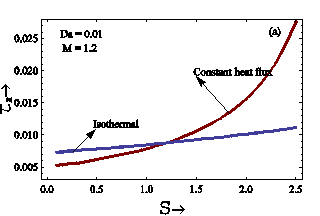## 9b.pngFigure 9. Skin-friction of the external cylinder at R=2.0. (a) with different values of Source and (b) with different values of Sink

Table 1. Skin friction and volume flow rate for Da=0.01, M=1.2

 S R τ1 τR τ1 τR Q Isothermal Constant heat flux Isothermal Constant heat flux 0.5 1.50 1.80 2.05 2.20 2.50 2.92 3.00 3.50 0.07193 0.08005 0.08359 0.08513 0.08754 0.09018 0.09064 0.09353 0.01514 0.00983 0.00742 0.00649 0.00529 0.00444 0.00435 0.00416 0.03020 0.05121 0.06923 0.08089 0.10807 0.16567 0.18200 0.45116 0.00636 0.00629 0.00614 0.00616 0.00653 0.00816 0.00874 0.02008 0.01006 0.02190 0.03322 0.04073 0.05777 0.08784 0.09468 0.15001 0.00422 0.01401 0.02752 0.03871 0.07133 0.16137 0.19011 0.72361 -0.5 1.50 1.80 2.05 2.20 2.50 2.92 3.00 3.50 0.07112 0.07830 0.08099 0.08202 0.08334 0.08434 0.08447 0.08498 0.01463 0.00892 0.00623 0.00515 0.00366 0.00239 0.00222 0.00141 0.02789 0.04270 0.05174 0.05603 0.06257 0.06843 0.06923 0.07270 0.00574 0.00487 0.00398 0.00352 0.00274 0.00194 0.00182 0.00121 0.00981 0.02056 0.02980 0.03533 0.04624 0.06070 0.06331 0.07836 0.00385 0.01121 0.01904 0.02414 0.03471 0.04925 0.05189 0.06705

## 1x8fzmmzrhuotsvmh9_mk.png## 10b.pngFigure 10. Volume flow rate at R=2.0. (a) with different values of Source and (b) with different values of Sink

The computational values of the skin-friction τ1 in the flow region of the internal cylinder, τR in the flow region of the external cylinder and the rate of mass flow are displayed in Table 1 in isothermal and uniform heat flux cases with source/sink parameter. From this table, we can notice that as the width of the duct increases, the skin friction at the internal surface increases while the skin friction at the external surface decreases in the case of isothermal boundary limitation. But in the case of uniform heat flux, the skin friction at the internal boundary represents the same nature while skin friction at the external boundary initially decreases for R≤2.05 and for larger values of R>2.05 the skin friction increases. In the case of the sink, the skin friction at the inner boundary presents similar behavior for isothermal and uniform heat flux while the skin friction at the outside cylinder reduces and there is no variation in the trend. Also, the dimensionless volume flow rate is directly proportional to the radii ratio in both cases.

4. Conclusions

The analytical solution of the supposed problem in phases of source/sink parameters, viscosity ratio, and Darcy number has been achieved. The influences of certain parameters such as the Darcy number on the fluid velocity, the temperature field, the skin-friction, and the dimensionless volume flow rate has been received. As a result of the relative comparison, we attained the following determinations:

(1) The temperature sketches are higher in the case of uniform heat flux than the isothermal circumstance for the source while the reversed aspect happens in the case of the sink.

(2) In the case of source, when the value of R<2.05, the velocity is greater for the isothermal case than the constant heat flux case, while the converse happening is noticed when R>2.05 for different values of Darcy number. The character of the velocity field sketches is almost the same in the situations of isothermal and uniform heat flux when the critical value of R=2.05 for the case of source (S=1.0).

(3) In the case of sink, for the value of R<2.92, the velocity is greater for isothermal case related to the uniform heat flux case, while the converse appearance has seen for distinguished values of Da when R>2.92. For R=2.92, the velocity fields are almost the same for both circumstances of isothermal and uniform heat flux with varying values of Da and M in the case of sink (Si=0.1).

(4) The velocity of the fluid enhances in the case of the source while it reduced in the case of sink though the impact of the viscosity ratio parameter is to minimize the velocity field sketches not only in the case of isothermal but also in the constant heat flux case.

(5) The skin-frictions at the cylinders and the dimensionless volume flow rate of a fluid between the cylinders are enhanced by raising the gap among the cylinders.

Nomenclature
 a&b Inner and Outer Radii S Heat Source in non-dimensional form Si Heat Sink in non-dimensional form $g$ Acceleration due to gravity ${K}'$ Permeability of the porous medium k Thermal conductivity ${u}'$ Velocity $U$ Dimensionless velocity ${r}'$ Radial coordinate $r$ Dimensionless Radial coordinate $q$ Constant heat flux ${T}'$ Temperature of the fluid T Non-dimensional temperature ${{{T}'}_{w}}$ Temperature of exterior surface of the interior cylinder in the case of isothermal heating ${{{T}'}_{\infty }}$ Free stream temperature ${{C}_{p}}$ Specific heat at a fixed pressure ${{I}_{n}}(r)$ Modified Bessel function of first kind of order n ${{K}_{n}}(r)$ Modified Bessel function of second kind of order n ${{J}_{n}}(r)$ Bessel function of first kind of order n ${{Y}_{n}}(r)$ Bessel function of second kind of order n Da Darcy number $Q$ Volumetric rate of heat generation/absorption Greek symbols $\rho$ Density of fluid $\beta$ Volumetric coefficient of the thermal expansion $\Theta$ Source/Sink $R$ Ratio of radii, $b/a$ $\mu$ Dynamic viscosity $\upsilon$ Kinematic viscosity M Ratio of viscosities $\tau$ Skin friction ${{\tau }_{1}}$ Skin-friction on the outer surface of the inner cylinder ${{\tau }_{R}}$ Skin-friction on the inner surface of the outer cylinder
Appendix

Case 1: When source is present

${{C}_{1}}=-{{D}_{1}}\frac{{{Y}_{0}}\left( R\sqrt{S} \right)}{{{J}_{0}}\left( R\sqrt{S} \right)},$

${{C}_{2}}=\frac{{{E}_{3}}\left[ {{E}_{2}}{{K}_{0}}\left( \frac{1}{\sqrt{DaM}} \right)-{{E}_{1}}{{K}_{0}}\left( \frac{R}{\sqrt{DaM}} \right) \right]}{{{E}_{4}}},$

{{D}_{1}}=\frac{c{{J}_{0}}\left( R\sqrt{S} \right)}{\left\{ \left. \begin{align} & {{J}_{0}}\left( R\sqrt{S} \right)\left[ -\sqrt{S}{{Y}_{1}}\left( \sqrt{S} \right)a+b{{Y}_{0}}\left( \sqrt{S} \right) \right] \\ & -{{Y}_{0}}\left( R\sqrt{S} \right)\left[ -\sqrt{S}{{J}_{1}}\left( \sqrt{S} \right)a+b{{J}_{0}}\left( \sqrt{S} \right) \right] \\ \end{align} \right\} \right.},

${{D}_{2}}=\frac{-{{E}_{3}}\left[ {{E}_{2}}{{I}_{0}}\left( \frac{1}{\sqrt{DaM}} \right)-{{E}_{1}}{{I}_{0}}\left( \frac{R}{\sqrt{DaM}} \right) \right]}{{{E}_{4}}},$

${{E}_{1}}={{C}_{1}}{{J}_{0}}\left( \sqrt{S} \right)+{{D}_{1}}{{Y}_{0}}\left( \sqrt{S} \right),$

${{E}_{2}}={{C}_{1}}{{J}_{0}}\left( R\sqrt{S} \right)+{{D}_{1}}{{Y}_{0}}\left( R\sqrt{S} \right),$

${{E}_{3}}=\frac{Da}{\left( SDaM+1 \right)},$

\begin{align} & {{E}_{4}}={{I}_{0}}\left( \frac{1}{\sqrt{DaM}} \right){{K}_{0}}\left( \frac{R}{\sqrt{DaM}} \right) \\ & \quad \quad \quad \quad -{{K}_{0}}\left( \frac{1}{\sqrt{DaM}} \right){{I}_{0}}\left( \frac{R}{\sqrt{DaM}} \right), \\ \end{align}

${{F}_{1}}={{C}_{1}}{{J}_{1}}\left( \sqrt{S} \right)+{{D}_{1}}{{Y}_{1}}\left( \sqrt{S} \right),$

${{F}_{2}}={{C}_{1}}{{J}_{1}}\left( R\sqrt{S} \right)+{{D}_{1}}{{Y}_{1}}\left( R\sqrt{S} \right).$

Case 2: When sink is present

${{A}_{1}}=-{{B}_{1}}\frac{{{K}_{0}}\left( R\sqrt{Si} \right)}{{{I}_{0}}\left( R\sqrt{Si} \right)},$

${{A}_{2}}=\frac{{{P}_{3}}\left[ {{P}_{1}}{{K}_{0}}\left( \frac{R}{\sqrt{DaM}} \right)-{{P}_{2}}{{K}_{0}}\left( \frac{1}{\sqrt{DaM}} \right) \right]}{{{E}_{4}}},$

{{B}_{1}}=\frac{c{{I}_{0}}\left( R\sqrt{Si} \right)}{\left\{ \left. \begin{align} & {{I}_{0}}\left( R\sqrt{Si} \right)\left[ -\sqrt{Si}{{K}_{1}}\left( \sqrt{Si} \right)a+b{{K}_{0}}\left( \sqrt{Si} \right) \right] \\ & -{{K}_{0}}\left( R\sqrt{Si} \right)\left[ \sqrt{Si}{{I}_{1}}\left( \sqrt{Si} \right)a+b{{I}_{0}}\left( \sqrt{Si} \right) \right] \\ \end{align} \right\} \right.},

${{B}_{2}}=\frac{-{{P}_{3}}\left[ {{P}_{1}}{{I}_{0}}\left( \frac{R}{\sqrt{DaM}} \right)-{{P}_{2}}{{I}_{0}}\left( \frac{1}{\sqrt{DaM}} \right) \right]}{{{E}_{4}}},$

${{P}_{1}}={{A}_{1}}{{I}_{0}}\left( \sqrt{Si} \right)+{{B}_{1}}{{K}_{0}}\left( \sqrt{Si} \right),$

${{P}_{2}}={{A}_{1}}{{I}_{0}}\left( R\sqrt{Si} \right)+{{B}_{1}}{{K}_{0}}\left( R\sqrt{Si} \right),$

${{P}_{3}}=\frac{Da}{\left( SiDaM-1 \right)},$

${{F}_{3}}={{A}_{1}}{{I}_{1}}\left( \sqrt{Si} \right)-{{B}_{1}}{{K}_{1}}\left( \sqrt{Si} \right),$

${{F}_{4}}={{A}_{1}}{{I}_{1}}\left( R\sqrt{Si} \right)-{{B}_{1}}{{K}_{1}}\left( R\sqrt{Si} \right).$

References

 Ostrach, S. (1988). Natural convection in enclosures. Journal of Heat Transfer, 110(4b): 1175-1190. https://doi.org/10.1115/1.3250619

 Anurag, Singh, A.K., Chandran, P., Sacheti, N.C. (2020). Effect of Newtonian heating/cooling on free convection in an annular permeable region in the presence of heat source/sink. Heat Transfer, 1-21. https://doi.org/10.1002/htj.21900

 Majdi, H.S., Abdulkadhim, A., Abed, A.M. (2019). Numerical investigation of natural convection heat transfer in a parallelogramic enclosure having an inner circular cylinder using liquid nanofluid. Frointers Heat Mass Transf, 12(2): 1-14.

 Abdulkadhim, A., Abed, A.M., Mohsen, A.M., AlFarhany, K. (2018). Effect of partially thermally active wall on natural convection in porous enclosure. Mathematical Modelling of Engineering Problems, 5(4): 395-406.

 Khanafer, K.M., Chamkha, A.J. (1998). Hydromagnetic natural convection from an inclined porous square enclosure with heat generation. Numerical Heat Transfer, Part A Applications, 33(8): 891-910. https://doi.org/10.1080/10407789808913972

 Baytas, A.C., Pop, I. (1999). Free convection in oblique enclosures filled with a porous medium. International Journal of Heat and Mass Transfer, 42(6): 1047-1057. https://doi.org/10.1016/S0017-9310(98)00208-7

 Al-Amiri, A.M. (2002). Natural convection in porous enclosures: the application of the two-energy equation model. Numerical Heat Transfer: Part A: Applications, 41(8): 817-834. https://doi.org/10.1080/10407780290059369

 Ingham, D.B., Pop, I. (1998). Transport Phenomena in Porous Media. Elsevier. https://doi.org/10.1016/B978-0-08-042843-7.X5000-4

 Vafai, K. (2005). Handbook of Porous Media. 2nd edn. Taylor & Francis, Boca Raton.

 Pop, I., Ingham, D.B. (2001). Convective Heat Transfer: Mathematical and Computational Modelling of Viscous Fluids and Porous Media. Elsevier.

 Vadesz, P. (2008). Emerging Topics in Heat and Mass Transfer in Porous Media. Springer, New York.

 Nield, D.A., Bejan, A. (2013). Convection in Porous Media. Springer, New York.

 Maurya, J.P., Yadav, S.L., Singh, A.K. (2020). Analysis of magnetohydrodynamics transient flow in a horizontal annular duct. International Journal of Dynamics and Control, 8: 730-740.

 Oni, M.O. (2017). Combine effect of heat source, porosity and thermal radiation on mixed convection flow in a vertical annulus: An exact solution. Engineering Science and Technology, 20: 518-527.

 Vafai, K., Tien, C.L. (1981). Boundary and inertia effects on flow and heat transfer in porous media. International Journal of Heat and Mass Transfer, 24(2): 195-203. https://doi.org/10.1016/0017-9310(81)90027-2

 Ranganathan, P., Viskanta, R. (1984). Mixed convection boundary-layer flow along a vertical surface in a porous medium. Numerical Heat Transfer, 7(3): 305-317. https://doi.org/10.1080/01495728408961827

 Aung, W. (1972). Fully developed laminar free convection between vertical plates heated asymmetrically. International Journal of Heat and Mass Transfer, 15(8): 1577-1580. https://doi.org/10.1016/0017-9310(72)90012-9

 Nelson, D.J., Wood, B.D. (1989). Fully developed combined heat and mass transfer natural convection between vertical parallel plates with symmetric boundary conditions. International Journal of Heat and Mass Transfer, 33: 1789-1792.

 Joshi, H.M. (1987). Fully developed natural convection in an isothermal vertical annular duct. International Communications in Heat and Mass Transfer, 14(6): 657-664. https://doi.org/10.1016/0735-1933(87)90045-5

 Paul, T., Singh, A.K. (1998). Natural convection between coaxial vertical cylinders partially filled with a porous material. Forschung im Ingenieurwesen, 64(6-7): 157-162. https://doi.org/10.1007/PL00010772

 Tong, T.W., Subramanian, E. (1985). A boundary-layer analysis for natural convection in vertical porous enclosures-Use of the Brinkman-extended Darcy model. International Journal of Heat and Mass Transfer, 28(3): 563-571. https://doi.org/10.1016/0017-9310(85)90179-6

 Lauriat, G., Prasad, V. (1986). Natural convection in a vertical porous cavity: A numerical study for Brinkmann extended Darcy formulation. Journal of Heat Transfer, 109(3): 688-696. https://doi.org/10.1115/1.3248143

 Parang, M., Keyhani, M. (1987). Boundary effects in laminar mixed convection flow through an annular porous medium. Journal of Heat Transfer (Transactions of the ASME (American Society of Mechanical Engineers), Series C) (United States), 109(4): 1039-1041. https://doi.org/10.1115/1.3248179

 Prasad, V., Kulacki, F.A., Kulkarni, A.V. (1986). Free convection in a vertical, porous annulus with constant heat flux on the inner wall-experimental results. International Journal of Heat and Mass Transfer, 29(5): 713-723. https://doi.org/10.1016/0017-9310(86)90123-7

 Havstad, M.A., Burns, P.J. (1982). Convective heat transfer in vertical cylindrical annuli filled with a porous medium. International Journal of Heat and Mass Transfer, 25(11): 1755-1766. https://doi.org/10.1016/0017-9310(82)90155-7

 Muralidhar, K., Kulacki, F.A. (1988). Non-Darcy natural convection in a saturated horizontal porous annulus. The American Society of Mechanical Engineers, Journal Heat Transfer, 110(1): 133-139. https://doi.org/10.1115/1.3250443

 Kaviany, M. (1986). Non-Darcian effects on natural convection in porous media confined between horizontal cylinders. International Journal of Heat and Mass Transfer, 29(10): 1513-1519. https://doi.org/10.1016/0017-9310(86)90066-9

 Khanafer, K., Chamkha, A.J. (2003). Mixed convection within a porous heat generating horizontal annulus. International Journal of Heat and Mass Transfer, 46(10): 1725-1735. https://doi.org/10.1016/S0017-9310(02)00483-0

 Murty, V.D., Clay, C.L., Camden, M.P., Paul, D.B. (1989). Natural convection in porous media in a cylindrical annulus–effect of radius ratio. In Proceedings of the Int Conf on Numerical Methods, pp. 487-496.

 Bejan, A., Tien, C.L. (1979). Natural convection in horizontal space bounded by two concentric cylinders with different end temperatures. International Journal of Heat and Mass Transfer, 22(6): 919-927. https://doi.org/10.1016/0017-9310(79)90032-2

 Singh, S.K., Jha, B.K., Singh, A.K. (1997). Natural convection in vertical concentric annuli under a radial magnetic field. Heat and Mass Transfer, 32(5): 399-401. https://doi.org/10.1007/s002310050137

 Yusuf, T.S. (2017). Exact solution of an MHD natural convection flow in vertical concentric annulus with heat absorption. International Journal of Fluid Mechanics and Thermal Sciences, 3(5): 52-61.

 Rapits, A., Takhar, H.S. (1987). Flow through porous medium. Mechanical Research Communication, 14: 327-329.

 Cheng, C.Y. (2006). The effect of temperature-dependent viscosity on the natural convection heat transfer from a horizontal isothermal cylinder of elliptic cross section. International Communications in Heat and Mass Transfer, 33(8): 1021-1028. https://doi.org/10.1016/j.icheatmasstransfer.2006.02.019

 Cheng, C.Y. (2009). Natural convection heat transfer from a horizontal isothermal elliptical cylinder with internal heat generation. International Communications in Heat and Mass Transfer, 36(4): 346-350.

 Molla, M.M., Hossain, M.A., Paul, M.C. (2006). Natural convection flow from an isothermal horizontal circular cylinder in presence of heat generation. International Journal of Engineering Science, 44: 949-958. https://doi.org/10.1016/j.icheatmasstransfer.2009.01.004

 Ravi, S.K., Singh, A.K. (2011). Natural convection of a polar fluid in vertical concentric annuli. International Journal of Applied Mathematics and Mechanics, 7: 70-81.

 Yadav, S.L., Singh, A.K. (2016). Analysis of entropy generation in annular porous duct. Transport in Porous Media, 111(2): 425-440. https://doi.org/10.1007/s11242-015-0602-x

 Jha, B.K. (2005). Free-convection flow through an annular porous medium. Heat and Mass Transfer, 41(8): 675-679. https://doi.org/10.1007/s00231-003-0469-1

 Ravi, S.K., Singh, A.K., Alawadhi, K.A. (2011). Effect of temperature dependent heat source/sink on free convective flow of a micropolar fluid between two vertical walls. International Journal of Energy and Technology, 3: 1-8.

 Singh, A.K., Singh, A.K. (2017). Effect of heat source/sink on free convective flow of a polar fluid between vertical concentric annuli. Journal of Applied Mathematics and Physics, 5: 1750-1762. https://www.scirp.org/html/6-1720918_79183.htm

 Kumar, D., Singh, A.K. (2016). Effects of heat source/sink and induced magnetic field on natural convective flow in vertical concentric annuli. Alexandria Engineering Journal, 55(4): 3125-3133. https://doi.org/10.1016/j.aej.2016.08.019

 Yadav, S.L., Singh, A.K. (2019). Analysis of entropy generation in a horizontal channel containing fluid and porous layers. Journal of Porous Media, 22(10): 1259-1271.

 Merkin, J.H. (1994). Natural-convection boundary-layer flow on a vertical surface with Newtonian heating. International Journal of Heat and Fluid Flow, 15(5): 392-398. https://doi.org/10.1016/0142-727X(94)90053-1

 Kumar, A., Singh, A.K. (2013). Effect of induced magnetic field on natural convection in vertical concentric annuli heated/cooled asymmetrically. Journal of Applied Fluid Mechanics, 6(1): 15-26.

 Yadav, S.L., Singh, A.K. (2014). Effects of viscous and Darcy dissipations on entropy generation rate of flow through a horizontal porous channel. International Journal of Energy & Technology, 6(17): 1-7.

 Kumar, D., Singh, A.K., Kumar, D. (2019). Influence of heat source/sink on MHD flow between vertical alternate conducting walls with Hall effect. Physica A: Statistical Mechanics and Its Applications, 544: 1-22. https://doi.org/10.1016/j.physa.2019.123562

 Dwivedi, N., Singh, A.K., Kumar, A. (2019). Natural convection between vertical walls due to point/line heat source/sink. International Journal of Applied and Computational Mathematics, 5: 75. https://doi.org/10.1007/s40819-019-0659-2